$$\require{cancel}$$

# 6.1: Free Waves

•• Contributed by Benjamin Crowell
• Professor (Physics) at Fullerton College

# 6.1.1 Wave motion

Let's start with an intuition-building exercise that deals with waves in matter, since they're easier than light waves to get your hands on. Put your fingertip in the middle of a cup of water and then remove it suddenly. You'll have noticed two results that are surprising to most people. First, the flat surface of the water does not simply sink uniformly to fill in the volume vacated by your finger. Instead, ripples spread out, and the process of flattening out occurs over a long period of time, during which the water at the center vibrates above and below the normal water level. This type of wave motion is the topic of the present section. Second, you've found that the ripples bounce off of the walls of the cup, in much the same way that a ball would bounce off of a wall. In the next section we discuss what happens to waves that have a boundary around them. Until then, we confine ourselves to wave phenomena that can be analyzed as if the medium (e.g., the water) was infinite and the same everywhere.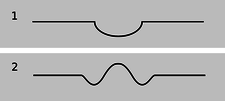Figure a: Your finger makes a depression in the surface of the water, 1. The wave patterns starts evolving, 2, after you remove your finger.

It isn't hard to understand why removing your fingertip creates ripples rather than simply allowing the water to sink back down uniformly. The initial crater, a/1, left behind by your finger has sloping sides, and the water next to the crater flows downhill to fill in the hole. The water far away, on the other hand, initially has no way of knowing what has happened, because there is no slope for it to flow down. As the hole fills up, the rising water at the center gains upward momentum, and overshoots, creating a little hill where there had been a hole originally. The area just outside of this region has been robbed of some of its water in order to build the hill, so a depressed “moat” is formed, a/2. This effect cascades outward, producing ripples.

There are three main ways in which wave motion differs from the motion of objects made of matter.Figure b: The two circular patterns of ripples pass through each other. Unlike material objects, wave patterns can overlap in space, and when this happens they combine by addition.

## 1. Superposition

If you watched the water in the cup carefully, you noticed the ghostlike behavior of the reflected ripples coming back toward the center of the cup and the outgoing ripples that hadn't yet been reflected: they passed right through each other. This is the first, and the most profound, difference between wave motion and the motion of objects: waves do not display any repulsion of each other analogous to the normal forces between objects that come in contact. Two wave patterns can therefore overlap in the same region of space, as shown in figure b. Where the two waves coincide, they add together. For instance, suppose that at a certain location in at a certain moment in time, each wave would have had a crest 3 cm above the normal water level. The waves combine at this point to make a 6-cm crest. We use negative numbers to represent depressions in the water. If both waves would have had a troughs measuring $$-3$$ cm, then they combine to make an extra-deep $$-6$$ cm trough. A $$+3$$ cm crest and a $$-3$$ cm trough result in a height of zero, i.e., the waves momentarily cancel each other out at that point. This additive rule is referred to as the principle of superposition, “superposition” being merely a fancy word for “adding.”

Superposition can occur not just with sinusoidal waves like the ones in the figure above but with waves of any shape. The figures on the following page show superposition of wave pulses. A pulse is simply a wave of very short duration. These pulses consist only of a single hump or trough. If you hit a clothesline sharply, you will observe pulses heading off in both directions. This is analogous to the way ripples spread out in all directions when you make a disturbance at one point on water. The same occurs when the hammer on a piano comes up and hits a string.

Experiments to date have not shown any deviation from the principle of superposition in the case of light waves. For other types of waves, it is typically a very good approximation for low-energy waves.c / As the wave pattern passes the rubber duck, the duck stays put. The water isn't moving with the wave.

## 2. The medium is not transported with the wave.

The sequence of three photos in figure c shows a series of water waves before it has reached a rubber duck (left), having just passed the duck (middle) and having progressed about a meter beyond the duck (right). The duck bobs around its initial position, but is not carried along with the wave. This shows that the water itself does not flow outward with the wave. If it did, we could empty one end of a swimming pool simply by kicking up waves! We must distinguish between the motion of the medium (water in this case) and the motion of the wave pattern through the medium. The medium vibrates; the wave progresses through space.

self-check:

In figure d, you can detect the side-to-side motion of the spring because the spring appears blurry. At a certain instant, represented by a single photo, how would you describe the motion of the different parts of the spring? Other than the flat parts, do any parts of the spring have zero velocity? (answer in the back of the PDF version of the book)Figure d: As the wave pulse goes by, the ribbon tied to the spring is not carried along. The motion of the wave pattern is to the right, but the medium (spring) is moving from side to side, not to the right. (PSSC Physics)

Example 1: A worm

The worm in the figure is moving to the right. The wave pattern, a pulse consisting of a compressed area of its body, moves to the left. In other words, the motion of the wave pattern is in the opposite direction compared to the motion of the medium.Example 2: Surfing

The incorrect belief that the medium moves with the wave is often reinforced by garbled secondhand knowledge of surfing. Anyone who has actually surfed knows that the front of the board pushes the water to the sides, creating a wake --- the surfer can even drag his hand through the water, as in in figure e. If the water was moving along with the wave and the surfer, this wouldn't happen. The surfer is carried forward because forward is downhill, not because of any forward flow of the water. If the water was flowing forward, then a person floating in the water up to her neck would be carried along just as quickly as someone on a surfboard. In fact, it is even possible to surf down the back side of a wave, although the ride wouldn't last very long because the surfer and the wave would quickly part company.e / Example 2. The surfer is dragging his hand in the water.

## 3. A wave's velocity depends on the medium

A material object can move with any velocity, and can be sped up or slowed down by a force that increases or decreases its kinetic energy. Not so with waves. The speed of a wave, depends on the properties of the medium (and perhaps also on the shape of the wave, for certain types of waves). Sound waves travel at about 340 m/s in air, 1000 m/s in helium. If you kick up water waves in a pool, you will find that kicking harder makes waves that are taller (and therefore carry more energy), not faster. The sound waves from an exploding stick of dynamite carry a lot of energy, but are no faster than any other waves. In the following section we will give an example of the physical relationship between the wave speed and the properties of the medium.

Example 3: Breaking waves

The velocity of water waves increases with depth. The crest of a wave travels faster than the trough, and this can cause the wave to break.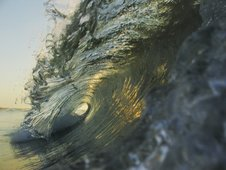Figure f: A breaking wave.

Once a wave is created, the only reason its speed will change is if it enters a different medium or if the properties of the medium change. It is not so surprising that a change in medium can slow down a wave, but the reverse can also happen. A sound wave traveling through a helium balloon will slow down when it emerges into the air, but if it enters another balloon it will speed back up again! Similarly, water waves travel more quickly over deeper water, so a wave will slow down as it passes over an underwater ridge, but speed up again as it emerges into deeper water.

Example 4: Hull speed

The speeds of most boats, and of some surface-swimming animals, are limited by the fact that they make a wave due to their motion through the water. The boat in figure g is going at the same speed as its own waves, and can't go any faster. No matter how hard the boat pushes against the water, it can't make the wave move ahead faster and get out of the way. The wave's speed depends only on the medium. Adding energy to the wave doesn't speed it up, it just increases its amplitude.Figure g: The boat has run up against a limit on its speed because it can't climb over its own wave. Dolphins get around the problem by leaping out of the water.

A water wave, unlike many other types of wave, has a speed that depends on its shape: a broader wave moves faster. The shape of the wave made by a boat tends to mold itself to the shape of the boat's hull, so a boat with a longer hull makes a broader wave that moves faster. The maximum speed of a boat whose speed is limited by this effect is therefore closely related to the length of its hull, and the maximum speed is called the hull speed. Sailboats designed for racing are not just long and skinny to make them more streamlined --- they are also long so that their hull speeds will be high.

## Wave patterns

If the magnitude of a wave's velocity vector is preordained, what about its direction? Waves spread out in all directions from every point on the disturbance that created them. If the disturbance is small, we may consider it as a single point, and in the case of water waves the resulting wave pattern is the familiar circular ripple, h/1. If, on the other hand, we lay a pole on the surface of the water and wiggle it up and down, we create a linear wave pattern, h/2. For a three-dimensional wave such as a sound wave, the analogous patterns would be spherical waves and plane waves, i.Figure h: Circular and linear wave patterns.i / Plane and spherical wave patterns.

Infinitely many patterns are possible, but linear or plane waves are often the simplest to analyze, because the velocity vector is in the same direction no matter what part of the wave we look at. Since all the velocity vectors are parallel to one another, the problem is effectively one-dimensional. Throughout this chapter and the next, we will restrict ourselves mainly to wave motion in one dimension, while not hesitating to broaden our horizons when it can be done without too much complication.

### Discussion Questions

◊ The left panel of the figure shows a sequence of snapshots of two positive pulses on a coil spring as they move through each other. In the right panel, which shows a positive pulse and a negative one, the fifth frame has the spring just about perfectly flat. If the two pulses have essentially canceled each other out perfectly, then why does the motion pick up again? Why doesn't the spring just stay flat?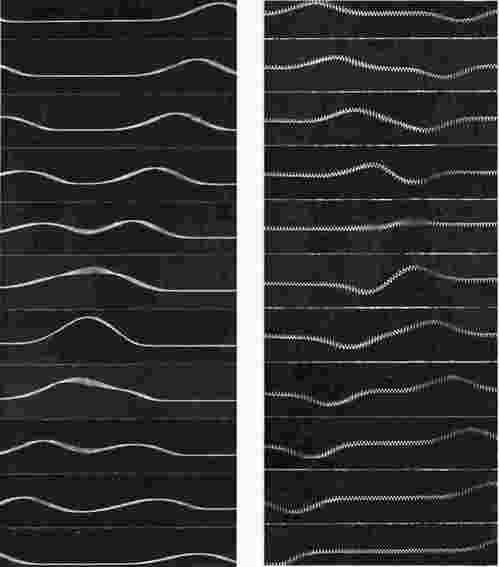j / Discussion question A.

◊ Sketch two positive wave pulses on a string that are overlapping but not right on top of each other, and draw their superposition. Do the same for a positive pulse running into a negative pulse.

◊ A traveling wave pulse is moving to the right on a string. Sketch the velocity vectors of the various parts of the string. Now do the same for a pulse moving to the left.

◊ In a spherical sound wave spreading out from a point, how would the energy of the wave fall off with distance?

# 6.1.2 Waves on a stringk / Hitting a key on a piano causes a hammer to come up from underneath and hit a string (actually a set of three). The result is a pair of pulses moving away from the point of impact.

So far you've learned some counterintuitive things about the behavior of waves, but intuition can be trained. The first half of this subsection aims to build your intuition by investigating a simple, one-dimensional type of wave: a wave on a string. If you have ever stretched a string between the bottoms of two open-mouthed cans to talk to a friend, you were putting this type of wave to work. Stringed instruments are another good example. Although we usually think of a piano wire simply as vibrating, the hammer actually strikes it quickly and makes a dent in it, which then ripples out in both directions. Since this chapter is about free waves, not bounded ones, we pretend that our string is infinitely long.

After the qualitative discussion, we will use simple approximations to investigate the speed of a wave pulse on a string. This quick and dirty treatment is then followed by a rigorous attack using the methods of calculus, which turns out to be both simpler and more general.

## Intuitive ideas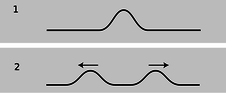l / A pulse on a string splits in two and heads off in both directions.

Consider a string that has been struck, l/1, resulting in the creation of two wave pulses, l/2, one traveling to the left and one to the right. This is analogous to the way ripples spread out in all directions from a splash in water, but on a one-dimensional string, “all directions” becomes “both directions.”Figure m: Modeling a string as a series of masses connected by springs.

We can gain insight by modeling the string as a series of masses connected by springs, m. (In the actual string the mass and the springiness are both contributed by the molecules themselves.) If we look at various microscopic portions of the string, there will be some areas that are flat, 1, some that are sloping but not curved, 2, and some that are curved, 3 and 4. In example 1 it is clear that both the forces on the central mass cancel out, so it will not accelerate. The same is true of 2, however. Only in curved regions such as 3 and 4 is an acceleration produced. In these examples, the vector sum of the two forces acting on the central mass is not zero. The important concept is that curvature makes force: the curved areas of a wave tend to experience forces resulting in an acceleration toward the mouth of the curve. Note, however, that an uncurved portion of the string need not remain motionless. It may move at constant velocity to either side.

## Approximate treatment

We now carry out an approximate treatment of the speed at which two pulses will spread out from an initial indentation on a string. For simplicity, we imagine a hammer blow that creates a triangular dent, n/1. We will estimate the amount of time, $$t$$, required until each of the pulses has traveled a distance equal to the width of the pulse itself. The velocity of the pulses is then $$\pm w/t$$.n / A triangular pulse spreads out.

As always, the velocity of a wave depends on the properties of the medium, in this case the string. The properties of the string can be summarized by two variables: the tension, $$T$$, and the mass per unit length, $$\mu$$ (Greek letter mu).

If we consider the part of the string encompassed by the initial dent as a single object, then this object has a mass of approximately $$\mu w$$ (mass/length $$\times\ \text{length}$$=mass). (Here, and throughout the derivation, we assume that $$h$$ is much less than $$w$$, so that we can ignore the fact that this segment of the string has a length slightly greater than $$w$$.) Although the downward acceleration of this segment of the string will be neither constant over time nor uniform across the pulse, we will pretend that it is constant for the sake of our simple estimate. Roughly speaking, the time interval between n/1 and n/2 is the amount of time required for the initial dent to accelerate from rest and reach its normal, flattened position. Of course the tip of the triangle has a longer distance to travel than the edges, but again we ignore the complications and simply assume that the segment as a whole must travel a distance $$h$$. Indeed, it might seem surprising that the triangle would so neatly spring back to a perfectly flat shape. It is an experimental fact that it does, but our analysis is too crude to address such details.

The string is kinked, i.e., tightly curved, at the edges of the triangle, so it is here that there will be large forces that do not cancel out to zero. There are two forces acting on the triangular hump, one of magnitude $$T$$ acting down and to the right, and one of the same magnitude acting down and to the left. If the angle of the sloping sides is $$\theta$$, then the total force on the segment equals $$2T \sin\theta$$. Dividing the triangle into two right triangles, we see that $$\sin\theta$$ equals $$h$$ divided by the length of one of the sloping sides. Since $$h$$ is much less than $$w$$, the length of the sloping side is essentially the same as $$w/2$$, so we have $$\sin\theta = 2h/w$$, and $$F=4Th/w$$. The acceleration of the segment (actually the acceleration of its center of mass) is

\begin{align*} a &= \frac{F}{m} \\ &= \frac{4Th}{\mu w^2} . \end{align*}

The time required to move a distance $$h$$ under constant acceleration $$a$$ is found by solving $$h=(1/2)at^2$$ to yield

\begin{align*} t &= \sqrt{2h/a} \\ &= w \sqrt{\frac{\mu}{2T}} . \end{align*}

Our final result for the speed of the pulses is

\begin{align*} v &= w/t\\ &= \sqrt{\frac{2T}{\mu}} . \end{align*}

The remarkable feature of this result is that the velocity of the pulses does not depend at all on $$w$$ or $$h$$, i.e., any triangular pulse has the same speed. It is an experimental fact (and we will also prove rigorously below) that any pulse of any kind, triangular or otherwise, travels along the string at the same speed. Of course, after so many approximations we cannot expect to have gotten all the numerical factors right. The correct result for the speed of the pulses is

$\begin{equation*} v = \sqrt{\frac{T}{\mu}} . \end{equation*}$

The importance of the above derivation lies in the insight it brings ---that all pulses move with the same speed --- rather than in the details of the numerical result. The reason for our too-high value for the velocity is not hard to guess. It comes from the assumption that the acceleration was constant, when actually the total force on the segment would diminish as it flattened out.

## Treatment using calculus

After expending considerable effort for an approximate solution, we now display the power of calculus with a rigorous and completely general treatment that is nevertheless much shorter and easier. Let the flat position of the string define the $$x$$ axis, so that $$y$$ measures how far a point on the string is from equilibrium. The motion of the string is characterized by $$y(x,t)$$, a function of two variables. Knowing that the force on any small segment of string depends on the curvature of the string in that area, and that the second derivative is a measure of curvature, it is not surprising to find that the infinitesimal force $$dF$$ acting on an infinitesimal segment $$dx$$ is given by

$\begin{equation*} dF = T\:\frac{\partial^2y}{\partial x^2} dx . \end{equation*}$

(This can be proved by vector addition of the two infinitesimal forces acting on either side.) The symbol $$\partial$$ stands for a partial derivative, e.g., $$\partial/\partial x$$ means a derivative with respect to $$x$$ that is evaluated while treating $$t$$ as a constant. The acceleration is then $$a =dF/dm$$, or, substituting $$dm=\mu dx$$,

$\begin{equation*} \frac{\partial^2y}{\partial t^2} = \frac{T}{\mu}\:\frac{\partial^2y}{\partial x^2} . \end{equation*}$

The second derivative with respect to time is related to the second derivative with respect to position. This is no more than a fancy mathematical statement of the intuitive fact developed above, that the string accelerates so as to flatten out its curves.

Before even bothering to look for solutions to this equation, we note that it already proves the principle of superposition, because the derivative of a sum is the sum of the derivatives. Therefore the sum of any two solutions will also be a solution.

Based on experiment, we expect that this equation will be satisfied by any function $$y(x,t)$$ that describes a pulse or wave pattern moving to the left or right at the correct speed $$v$$. In general, such a function will be of the form $$y=f(x-vt)$$ or $$y=f(x+vt)$$, where $$f$$ is any function of one variable. Because of the chain rule, each derivative with respect to time brings out a factor of $$v$$. Evaluating the second derivatives on both sides of the equation gives

$\begin{equation*} (\pm v)^2\: f” = \frac{T}{\mu}\:f” . \end{equation*}$

Squaring gets rid of the sign, and we find that we have a valid solution for any function $$f$$, provided that $$v$$ is given by

$\begin{equation*} v = \sqrt{\frac{T}{\mu}} . \end{equation*}$

# 6.1.3 Sound and light waves

## Sound waves

The phenomenon of sound is easily found to have all the characteristics we expect from a wave phenomenon:

• Sound waves obey superposition. Sounds do not knock other sounds out of the way when they collide, and we can hear more than one sound at once if they both reach our ear simultaneously.
• The medium does not move with the sound. Even standing in front of a titanic speaker playing earsplitting music, we do not feel the slightest breeze.
• The velocity of sound depends on the medium. Sound travels faster in helium than in air, and faster in water than in helium. Putting more energy into the wave makes it more intense, not faster. For example, you can easily detect an echo when you clap your hands a short distance from a large, flat wall, and the delay of the echo is no shorter for a louder clap.

Although not all waves have a speed that is independent of the shape of the wave, and this property therefore is irrelevant to our collection of evidence that sound is a wave phenomenon, sound does nevertheless have this property. For instance, the music in a large concert hall or stadium may take on the order of a second to reach someone seated in the nosebleed section, but we do not notice or care, because the delay is the same for every sound. Bass, drums, and vocals all head outward from the stage at 340 m/s, regardless of their differing wave shapes. (The speed of sound in a gas is related to the gas's physical properties in example 13 on p. 375.)

If sound has all the properties we expect from a wave, then what type of wave is it? It is a series of compressions and expansions of the air. Even for a very loud sound, the increase or decrease compared to normal atmospheric pressure is no more than a part per million, so our ears are apparently very sensitive instruments. In a vacuum, there is no medium for the sound waves, and so they cannot exist. The roars and whooshes of space ships in Hollywood movies are fun, but scientifically wrong.

## Light waves

Entirely similar observations lead us to believe that light is a wave, although the concept of light as a wave had a long and tortuous history. It is interesting to note that Isaac Newton very influentially advocated a contrary idea about light. The belief that matter was made of atoms was stylish at the time among radical thinkers (although there was no experimental evidence for their existence), and it seemed logical to Newton that light as well should be made of tiny particles, which he called corpuscles (Latin for “small objects”). Newton's triumphs in the science of mechanics, i.e., the study of matter, brought him such great prestige that nobody bothered to question his incorrect theory of light for 150 years. One persuasive proof that light is a wave is that according to Newton's theory, two intersecting beams of light should experience at least some disruption because of collisions between their corpuscles. Even if the corpuscles were extremely small, and collisions therefore very infrequent, at least some dimming should have been measurable. In fact, very delicate experiments have shown that there is no dimming.

The wave theory of light was entirely successful up until the 20th century, when it was discovered that not all the phenomena of light could be explained with a pure wave theory. It is now believed that both light and matter are made out of tiny chunks which have both wave and particle properties. For now, we will content ourselves with the wave theory of light, which is capable of explaining a great many things, from cameras to rainbows.

If light is a wave, what is waving? What is the medium that wiggles when a light wave goes by? It isn't air. A vacuum is impenetrable to sound, but light from the stars travels happily through zillions of miles of empty space. Light bulbs have no air inside them, but that doesn't prevent the light waves from leaving the filament. For a long time, physicists assumed that there must be a mysterious medium for light waves, and they called it the ether (not to be confused with the chemical). Supposedly the ether existed everywhere in space, and was immune to vacuum pumps. The details of the story are more fittingly reserved for later in this course, but the end result was that a long series of experiments failed to detect any evidence for the ether, and it is no longer believed to exist. Instead, light can be explained as a wave pattern made up of electrical and magnetic fields.

# 6.1.4 Periodic waves

## Period and frequency of a periodic wave

You choose a radio station by selecting a certain frequency. We have already defined period and frequency for vibrations,

\begin{align*} T &= \text{period} = \text{seconds per cycle} \\ f &= \text{frequency} = 1/T = \text{cycles per second} \\ \omega &= \text{angular frequency} = 2\pi f= \text{radians per second} \end{align*}

but what do they signify in the case of a wave? We can recycle our previous definition simply by stating it in terms of the vibrations that the wave causes as it passes a receiving instrument at a certain point in space. For a sound wave, this receiver could be an eardrum or a microphone. If the vibrations of the eardrum repeat themselves over and over, i.e., are periodic, then we describe the sound wave that caused them as periodic. Likewise we can define the period and frequency of a wave in terms of the period and frequency of the vibrations it causes. As another example, a periodic water wave would be one that caused a rubber duck to bob in a periodic manner as they passed by it.

The period of a sound wave correlates with our sensory impression of musical pitch. A high frequency (short period) is a high note. The sounds that really define the musical notes of a song are only the ones that are periodic. It is not possible to sing a nonperiodic sound like “sh” with a definite pitch.

The frequency of a light wave corresponds to color. Violet is the high-frequency end of the rainbow, red the low-frequency end. A color like brown that does not occur in a rainbow is not a periodic light wave. Many phenomena that we do not normally think of as light are actually just forms of light that are invisible because they fall outside the range of frequencies our eyes can detect. Beyond the red end of the visible rainbow, there are infrared and radio waves. Past the violet end, we have ultraviolet, x-rays, and gamma rays.

## Graphs of waves as a function of positiono / A graph of pressure versus time for a periodic sound wave, the vowel “ah.”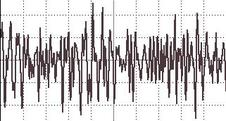p / A similar graph for a nonperiodic wave, “sh.”

Some waves, light sound waves, are easy to study by placing a detector at a certain location in space and studying the motion as a function of time. The result is a graph whose horizontal axis is time. With a water wave, on the other hand, it is simpler just to look at the wave directly. This visual snapshot amounts to a graph of the height of the water wave as a function of position. Any wave can be represented in either way.q / A strip chart recorder.

An easy way to visualize this is in terms of a strip chart recorder, an obsolescing device consisting of a pen that wiggles back and forth as a roll of paper is fed under it. It can be used to record a person's electrocardiogram, or seismic waves too small to be felt as a noticeable earthquake but detectable by a seismometer. Taking the seismometer as an example, the chart is essentially a record of the ground's wave motion as a function of time, but if the paper was set to feed at the same velocity as the motion of an earthquake wave, it would also be a full-scale representation of the profile of the actual wave pattern itself. Assuming, as is usually the case, that the wave velocity is a constant number regardless of the wave's shape, knowing the wave motion as a function of time is equivalent to knowing it as a function of position.r / A water wave profile created by a series of repeating pulses.

## Wavelength

Any wave that is periodic will also display a repeating pattern when graphed as a function of position. The distance spanned by one repetition is referred to as one wavelength. The usual notation for wavelength is $$\lambda$$, the Greek letter lambda. Wavelength is to space as period is to time.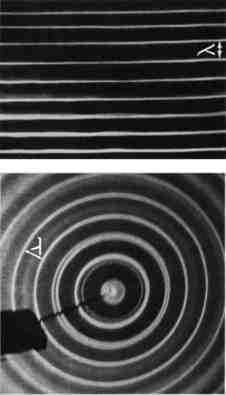s / Wavelengths of linear and circular waves.

## Wave velocity related to frequency and wavelength

Suppose that we create a repetitive disturbance by kicking the surface of a swimming pool. We are essentially making a series of wave pulses. The wavelength is simply the distance a pulse is able to travel before we make the next pulse. The distance between pulses is $$\lambda$$, and the time between pulses is the period, $$T$$, so the speed of the wave is the distance divided by the time,

$\begin{equation*} v = \lambda/T . \end{equation*}$

This important and useful relationship is more commonly written in terms of the frequency,

$\begin{equation*} v = f\lambda . \end{equation*}$

Example 5: length of radio waves

$$\triangleright$$ The speed of light is $$3.0\times10^8\ \text{m/s}$$. What is the wavelength of the radio waves emitted by KMHD, a station whose frequency is 89.1 MHz?

$$\triangleright$$ Solving for wavelength, we have

\begin{align*} \lambda &= v/ f \\ &= \text{(3.0}\times10^8\ \text{m/s) /(89.1}\times10^6\ \text{s}^{-1}) \ &= \text{3.4\ m} \end{align*}

The size of a radio antenna is closely related to the wavelength of the waves it is intended to receive. The match need not be exact (since after all one antenna can receive more than one wavelength!), but the ordinary “whip” antenna such as a car's is 1/4 of a wavelength. An antenna optimized to receive KMHD's signal would have a length of (3.4 m)/4 = 0.85 m.

The equation $$v=f \lambda$$ defines a fixed relationship between any two of the variables if the other is held fixed. The speed of radio waves in air is almost exactly the same for all wavelengths and frequencies (it is exactly the same if they are in a vacuum), so there is a fixed relationship between their frequency and wavelength. Thus we can say either “Are we on the same wavelength?” or “Are we on the same frequency?”

A different example is the behavior of a wave that travels from a region where the medium has one set of properties to an area where the medium behaves differently. The frequency is now fixed, because otherwise the two portions of the wave would otherwise get out of step, causing a kink or discontinuity at the boundary, which would be unphysical. (A more careful argument is that a kink or discontinuity would have infinite curvature, and waves tend to flatten out their curvature. An infinite curvature would flatten out infinitely fast, i.e., it could never occur in the first place.) Since the frequency must stay the same, any change in the velocity that results from the new medium must cause a change in wavelength.t / Ultrasound, i.e., sound with frequencies higher than the range of human hearing, was used to make this image of a fetus. The resolution of the image is related to the wavelength, since details smaller than about one wavelength cannot be resolved. High resolution therefore requires a short wavelength, corresponding to a high frequency.

The velocity of water waves depends on the depth of the water, so based on $$\lambda=v/f$$, we see that water waves that move into a region of different depth must change their wavelength, as shown in figure u.u / A water wave traveling into a region with different depth will change its wavelength.

This effect can be observed when ocean waves come up to the shore. If the deceleration of the wave pattern is sudden enough, the tip of the wave can curl over, resulting in a breaking wave.

## A note on dispersive waves

The discussion of wave velocity given here is actually a little bit of an oversimplification for a wave whose velocity depends on its frequency and wavelength. Such a wave is called a dispersive wave. Nearly all the waves we deal with in this course are nondispersive, but the issue becomes important in chapter 13, where it is discussed in detail.

## Sinusoidal waves

Sinusoidal waves are the most important special case of periodic waves. In fact, many scientists and engineers would be uncomfortable with defining a waveform like the “ah” vowel sound as having a definite frequency and wavelength, because they consider only sine waves to be pure examples of a certain frequency and wavelengths. Their bias is not unreasonable, since the French mathematician Fourier showed that any periodic wave with frequency $$f$$ can be constructed as a superposition of sine waves with frequencies $$f,\ 2f,\ 3f,\ ...$$ In this sense, sine waves are the basic, pure building blocks of all waves. (Fourier's result so surprised the mathematical community of France that he was ridiculed the first time he publicly presented his theorem.)

However, what definition to use is really a matter of convenience. Our sense of hearing perceives any two sounds having the same period as possessing the same pitch, regardless of whether they are sine waves or not. This is undoubtedly because our ear-brain system evolved to be able to interpret human speech and animal noises, which are periodic but not sinusoidal. Our eyes, on the other hand, judge a color as pure (belonging to the rainbow set of colors) only if it is a sine wave.

### Discussion Questions

Suppose we superimpose two sine waves with equal amplitudes but slightly different frequencies, as shown in the figure. What will the superposition look like? What would this sound like if they were sound waves?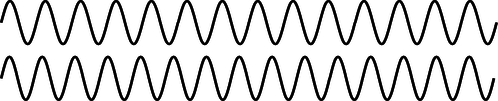v / Discussion question A.

# 6.1.5 The Doppler effectw / The pattern of waves made by a point source moving to the right across the water. Note the shorter wavelength of the forward-emitted waves and the longer wavelength of the backward-going ones.

Figure w shows the wave pattern made by the tip of a vibrating rod which is moving across the water. If the rod had been vibrating in one place, we would have seen the familiar pattern of concentric circles, all centered on the same point. But since the source of the waves is moving, the wavelength is shortened on one side and lengthened on the other. This is known as the Doppler effect.

Note that the velocity of the waves is a fixed property of the medium, so for example the forward-going waves do not get an extra boost in speed as would a material object like a bullet being shot forward from an airplane.

We can also infer a change in frequency. Since the velocity is constant, the equation $$v=f\lambda$$ tells us that the change in wavelength must be matched by an opposite change in frequency: higher frequency for the waves emitted forward, and lower for the ones emitted backward. The frequency Doppler effect is the reason for the familiar dropping-pitch sound of a race car going by. As the car approaches us, we hear a higher pitch, but after it passes us we hear a frequency that is lower than normal.

The Doppler effect will also occur if the observer is moving but the source is stationary. For instance, an observer moving toward a stationary source will perceive one crest of the wave, and will then be surrounded by the next crest sooner than she otherwise would have, because she has moved toward it and hastened her encounter with it. Roughly speaking, the Doppler effect depends only the relative motion of the source and the observer, not on their absolute state of motion (which is not a well-defined notion in physics) or on their velocity relative to the medium.

Restricting ourselves to the case of a moving source, and to waves emitted either directly along or directly against the direction of motion, we can easily calculate the wavelength, or equivalently the frequency, of the Doppler-shifted waves. Let $$u$$ be the velocity of the source. The wavelength of the forward-emitted waves is shortened by an amount $$uT$$ equal to the distance traveled by the source over the course of one period. Using the definition $$f=1/T$$ and the equation $$v=f\lambda$$, we find for the wavelength $$\lambda'$$ of the Doppler-shifted wave the equation

$\begin{equation*} \lambda' = \left(1-\frac{u}{v}\right)\:\lambda . \end{equation*}$

A similar equation can be used for the backward-emitted waves, but with a plus sign rather than a minus sign.

Example 6: Doppler-shifted sound from a race car

$$\triangleright$$ If a race car moves at a velocity of 50 m/s, and the velocity of sound is 340 m/s, by what percentage are the wavelength and frequency of its sound waves shifted for an observer lying along its line of motion?

$$\triangleright$$ For an observer whom the car is approaching, we find

$\begin{equation*} 1-\frac{ u}{ v}= 0.85 , \end{equation*}$

so the shift in wavelength is 15%. Since the frequency is inversely proportional to the wavelength for a fixed value of the speed of sound, the frequency is shifted upward by

$\begin{equation*} \text{1/0.85 = 1.18} , \end{equation*}$

i.e., a change of 18%. (For velocities that are small compared to the wave velocities, the Doppler shifts of the wavelength and frequency are about the same.)

Example 7: Doppler shift of the light emitted by a race car

$$\triangleright$$ What is the percent shift in the wavelength of the light waves emitted by a race car's headlights?

$$\triangleright$$ Looking up the speed of light in the back of the book, $$v= 3.0\times10^8\ \text{m/s}$$, we find

$\begin{equation*} 1-\frac{ u}{ v} \text{= 0.99999983} , \end{equation*}$

i.e., the percentage shift is only 0.000017%.

The second example shows that under ordinary earthbound circumstances, Doppler shifts of light are negligible because ordinary things go so much slower than the speed of light. It's a different story, however, when it comes to stars and galaxies, and this leads us to a story that has profound implications for our understanding of the origin of the universe.

## The Big Bang

As soon as astronomers began looking at the sky through telescopes, they began noticing certain objects that looked like clouds in deep space. The fact that they looked the same night after night meant that they were beyond the earth's atmosphere. Not knowing what they really were, but wanting to sound official, they called them “nebulae,” a Latin word meaning “clouds” but sounding more impressive. In the early 20th century, astronomers realized that although some really were clouds of gas (e.g., the middle “star” of Orion's sword, which is visibly fuzzy even to the naked eye when conditions are good), others were what we now call galaxies: virtual island universes consisting of trillions of stars (for example the Andromeda Galaxy, which is visible as a fuzzy patch through binoculars). Three hundred years after Galileo had resolved the Milky Way into individual stars through his telescope, astronomers realized that the universe is made of galaxies of stars, and the Milky Way is simply the visible part of the flat disk of our own galaxy, seen from inside.x / The galaxy M100 in the constellation Coma Berenices. Under higher magnification, the milky clouds reveal themselves to be composed of trillions of stars.

This opened up the scientific study of cosmology, the structure and history of the universe as a whole, a field that had not been seriously attacked since the days of Newton. Newton had realized that if gravity was always attractive, never repulsive, the universe would have a tendency to collapse. His solution to the problem was to posit a universe that was infinite and uniformly populated with matter, so that it would have no geometrical center. The gravitational forces in such a universe would always tend to cancel out by symmetry, so there would be no collapse. By the 20th century, the belief in an unchanging and infinite universe had become conventional wisdom in science, partly as a reaction against the time that had been wasted trying to find explanations of ancient geological phenomena based on catastrophes suggested by biblical events like Noah's flood.

In the 1920's astronomer Edwin Hubble began studying the Doppler shifts of the light emitted by galaxies. A former college football player with a serious nicotine addiction, Hubble did not set out to change our image of the beginning of the universe. His autobiography seldom even mentions the cosmological discovery for which he is now remembered. When astronomers began to study the Doppler shifts of galaxies, they expected that each galaxy's direction and velocity of motion would be essentially random. Some would be approaching us, and their light would therefore be Doppler-shifted to the blue end of the spectrum, while an equal number would be expected to have red shifts. What Hubble discovered instead was that except for a few very nearby ones, all the galaxies had red shifts, indicating that they were receding from us at a hefty fraction of the speed of light. Not only that, but the ones farther away were receding more quickly. The speeds were directly proportional to their distance from us.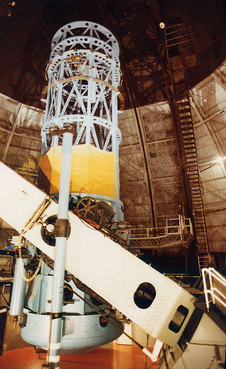y / The telescope at Mount Wilson used by Hubble.

Did this mean that the earth (or at least our galaxy) was the center of the universe? No, because Doppler shifts of light only depend on the relative motion of the source and the observer. If we see a distant galaxy moving away from us at 10% of the speed of light, we can be assured that the astronomers who live in that galaxy will see ours receding from them at the same speed in the opposite direction. The whole universe can be envisioned as a rising loaf of raisin bread. As the bread expands, there is more and more space between the raisins. The farther apart two raisins are, the greater the speed with which they move apart.

The universe's expansion is presumably decelerating because of gravitational attraction among the galaxies. We do not presently know whether there is enough mass in the universe to cause enough attraction to halt the expansion eventually. But perhaps more interesting than the distant future of the universe is what its present expansion implies about its past. Extrapolating backward in time using the known laws of physics, the universe must have been denser and denser at earlier and earlier times. At some point, it must have been extremely dense and hot, and we can even detect the radiation from this early fireball, in the form of microwave radiation that permeates space. The phrase Big Bang was originally coined by the doubters of the theory to make it sound ridiculous, but it stuck, and today essentially all astronomers accept the Big Bang theory based on the very direct evidence of the red shifts and the cosmic microwave background radiation.

Finally it should be noted what the Big Bang theory is not. It is not an explanation of why the universe exists. Such questions belong to the realm of religion, not science. Science can find ever simpler and ever more fundamental explanations for a variety of phenomena, but ultimately science takes the universe as it is according to observations.

Furthermore, there is an unfortunate tendency, even among many scientists, to speak of the Big Bang theory was a description of the very first event in the universe, which caused everything after it. Although it is true that time may have had a beginning (Einstein's theory of general relativity admits such a possibility), the methods of science can only work within a certain range of conditions such as temperature and density. Beyond a temperature of about $$10^9$$ K, the random thermal motion of subatomic particles becomes so rapid that its velocity is comparable to the speed of light. Early enough in the history of the universe, when these temperatures existed, Newtonian physics becomes less accurate, and we must describe nature using the more general description given by Einstein's theory of relativity, which encompasses Newtonian physics as a special case. At even higher temperatures, beyond about $$10^{33}$$ degrees, physicists know that Einstein's theory as well begins to fall apart, but we don't know how to construct the even more general theory of nature that would work at those temperatures. No matter how far physics progresses, we will never be able to describe nature at infinitely high temperatures, since there is a limit to the temperatures we can explore by experiment and observation in order to guide us to the right theory. We are confident that we understand the basic physics involved in the evolution of the universe starting a few minutes after the Big Bang, and we may be able to push back to milliseconds or microseconds after it, but we cannot use the methods of science to deal with the beginning of time itself.

## A note on Doppler shifts of light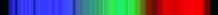z / How do astronomers know what mixture of wavelengths a star emitted originally, so that they can tell how much the Doppler shift was? This image (obtained by the author with equipment costing about \$5, and no telescope) shows the mixture of colors emitted by the star Sirius. (If you have the book in black and white, blue is on the left and red on the right.) The star appears white or bluish-white to the eye, but any light looks white if it contains roughly an equal mixture of the rainbow colors, i.e., of all the pure sinusoidal waves with wavelengths lying in the visible range. Note the black “gap teeth.” These are the fingerprint of hydrogen in the outer atmosphere of Sirius. These wavelengths are selectively absorbed by hydrogen. Sirius is in our own galaxy, but similar stars in other galaxies would have the whole pattern shifted toward the red end, indicating they are moving away from us.

If Doppler shifts depend only on the relative motion of the source and receiver, then there is no way for a person moving with the source and another person moving with the receiver to determine who is moving and who isn't. Either can blame the Doppler shift entirely on the other's motion and claim to be at rest herself. This is entirely in agreement with the principle stated originally by Galileo that all motion is relative.

On the other hand, a careful analysis of the Doppler shifts of water or sound waves shows that it is only approximately true, at low speeds, that the shifts just depend on the relative motion of the source and observer. For instance, it is possible for a jet plane to keep up with its own sound waves, so that the sound waves appear to stand still to the pilot of the plane. The pilot then knows she is moving at exactly the speed of sound. The reason this doesn't disprove the relativity of motion is that the pilot is not really determining her absolute motion but rather her motion relative to the air, which is the medium of the sound waves.

Einstein realized that this solved the problem for sound or water waves, but would not salvage the principle of relative motion in the case of light waves, since light is not a vibration of any physical medium such as water or air. Beginning by imagining what a beam of light would look like to a person riding a motorcycle alongside it, Einstein eventually came up with a radical new way of describing the universe, in which space and time are distorted as measured by observers in different states of motion. As a consequence of this Theory of Relativity, he showed that light waves would have Doppler shifts that would exactly, not just approximately, depend only on the relative motion of the source and receiver.

### Discussion Questionsaa / Shock waves are created by the X-15 rocket plane, flying at 3.5 times the speed of sound.ab / This fighter jet has just accelerated past the speed of sound. The sudden decompression of the air causes water droplets to condense, forming a cloud.

If an airplane travels at exactly the speed of sound, what would be the wavelength of the forward-emitted part of the sound waves it emitted? How should this be interpreted, and what would actually happen? What happens if it's going faster than the speed of sound? Can you use this to explain what you see in figures aa and ab?

◊ If bullets go slower than the speed of sound, why can a supersonic fighter plane catch up to its own sound, but not to its own bullets?

◊ If someone inside a plane is talking to you, should their speech be Doppler shifted?

# Contributors

Benjamin Crowell (Fullerton College). Conceptual Physics is copyrighted with a CC-BY-SA license.